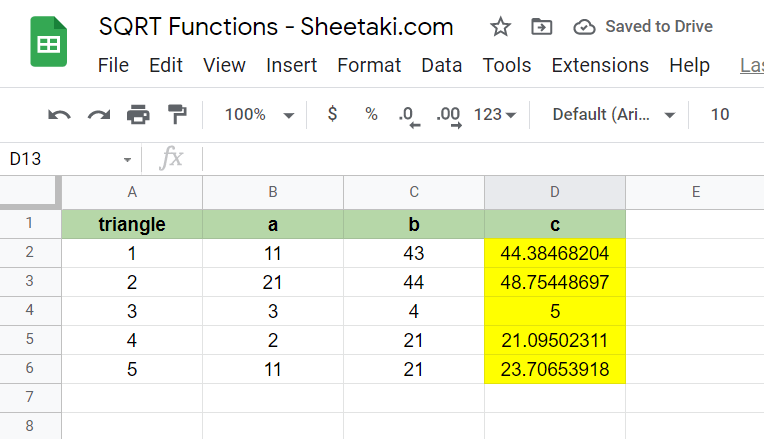# How To Use SQRT Function in Google Sheets

The SQRT function in Google Sheets is useful when you need to find the positive square root of a number.

The square root function is a common operation in mathematics. This particular function only works for positive numbers.

The rules for using the `SQRT` function in Google Sheets are as follows:

• The function requires a single argument. The `SQRT` function requires the number for which to calculate the positive square root.
• The output returned is the positive square root as a result.

Let’s take a look at an example of this function in use.

The Pythagorean Theorem is one of the most common formulas taught in the classroom. It’s needed in all types of fields, from physics to architecture. But for this particular example, we simply need to compute the diagonal of a grassy field.

We know that the field’s length is 175 m and that the width of the field is 95m. How do we solve for the diagonal?

From the Pythagorean theorem, we can derive the equation for solving the diagonal as c = a^2+b^2. Using the `SQRT` function, we can easily compute for the value of c.

This example is just one way we can use the `SQRT` function in Google Sheets. The square root is essential for so many equations, including equations in trigonometry, calculus, and more.

In the next section, let’s figure out how to write the `SQRT` function ourselves in Google Sheets.

## The Anatomy of the SQRT Function

The syntax of the `SQRT` function is as follows:

`=SQRT(value)`

Let’s dissect this thing and understand what each of these terms means:

• = the equal sign is how we start any type of function in Google Sheets.
• SQRT() is our `SQRT` function. It computes the positive square root of a number
• value refers to the number which we want to find the square root of.
• The value argument must be a positive number, else the function will return a #NUM! error

## A Real Example of Using SQRT Function

Let’s look into an example of the `SQRT` function being used in a Google Sheets spreadsheet.

In the worksheet below, we have typed in the length and width of our example earlier. Using the `SQRT` function in Google Sheets, we find out that the diagonal of the field is 199.123 m long.To get the values in cell B3, we just need to use the following formula:

```=SQRT(B1^2+B2^2)
```

In the second example below, we have values for sides a and b of multiple right triangles. Using the `SQRT` function, we’re able to find the values of the hypotenuse.If you would like to try out these worksheets yourself, you may use the link attached below to make your own copy.

Are you ready to try out the `SQRT` function in Google Sheets? Let’s begin writing it ourselves in the next section.

## How to Use SQRT Function in Google Sheets

In this step-by-step guide, we’ll be building the second example shown earlier.

1. We must first select the cell we will add our formula to first. In this example, we’ll start with cell D2.2. Next, we just have to type the equal sign ‘=‘ to start our function, followed by ‘SQRT(‘.
3. After typing in our function name, you may see a pop-up showing information about the function you just typed. If you would like to minimize this tooltip, you may click the arrow in the top-right-hand corner.4. The next step is to type our argument. Following the Pythagorean Theorem, we can use the following formula to get the first row’s result: =SQRT(B2^2 + C2^2)Afterward, simply hit Enter on your keyboard to let the function evaluate.
5. Finally, we can drag down the formula in cell D2 to fill out the rest of the table!1. How do I find out the square root of a negative number?
The `SQRT` function does not accept negative numbers as valid input. A possible workaround for this is to use the `ABS` function. The `ABS` function gets the absolute value of a given number. For example, `=ABS(-10)` is simply 10.
In the example below, we’ve wrapped our `SQRT` argument with an `ABS` function first in order to get the square root of a negative number.2. Is it possible to find the square root of a number without having to use the SQRT function?
It’s possible to find the square root of numbers by using the ‘^’ operator. We just need to raise the number to 0.5. For example, =100^0.5 is simply 10.

Hopefully, you now know more about the `SQRT` function in Google Sheets. This guide shows how easy it is to use the square root operation in Google Sheets.

You can now use the `SQRT` function in Google Sheets together with the various other Google Sheets formulas available to create powerful spreadsheets for any kind of computation.

If this article was informative, you can opt to stay notified of new guides like this by subscribing to our newsletter!Our goal this year is to create lots of rich, bite-sized tutorials for Google Sheets users like you. If you liked this one, you'd love what we are working on! Readers receive ✨ early access ✨ to new content.

##### You May Also Like## How to Limit Number of Rows in Google Sheets Query

The Query function in Google Sheets is a useful tool for looking up and filtering data onto your…## How to Use MINVERSE Function in Google Sheets

The MINVERSE function in Google Sheets is useful when you need to compute the multiplicative inverse of a…## How to Use the ERF.PRECISE Function in Google Sheets

The ERF.PRECISE function in Google Sheets calculates for the Gauss error function integrated between 0 and a given…## How to Merge Duplicate Rows in Google Sheets

To merge duplicate rows in Google Sheets seems complicated to me. There are times when I don’t really…